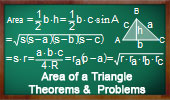# Plane Geometry: Area of a triangle, Theorems and Problems - Table of Content - Page 8Proposed Problem 85. Contact Triangles Areas, Incircle, Excircle.

Proposed Problem 84. Contact Triangles Areas, Incircle, Excircle, Inradius, Exradius.

Proposed Problem 83. Area of the Excircle Contact Triangle, exradius, circumradius.

De Gua's Theorem
Area of triangles.

Triangle and Squares 22
Area of triangles and rectangles.

Hippocrates and Squaring the Circle
Triangle areas

Heron's Formula with medians Triangle areas.

Kurschak's Tile and Theorem. Jozsef Kurschak (Hungary, 1864-1933) An elegant and a purely geometric way of finding the area of a regular dodecagon.
Triangle areas.

Euclid's Elements Book I, 23 Definitions. One-page visual illustration.
Euclid's Elements Book. Index

Varignon and Wittenbauer parallelograms. Quadrilateral: midpoints and trisection points of the edges. Triangle areas.

Go to Page: Previous | 1 | 2 | 3 | 4 | 5 | 6 | 7 | 8 | Next

 Home | Geometry | Areas | Email | Post a comment | By Antonio Gutierrez